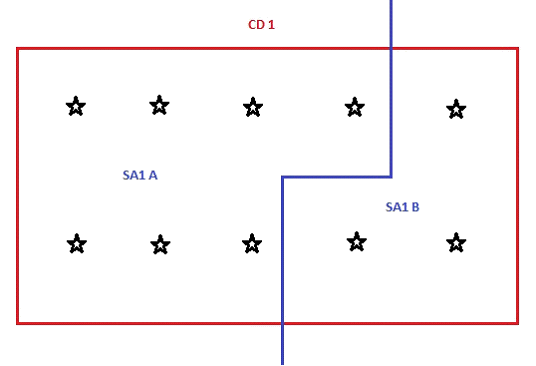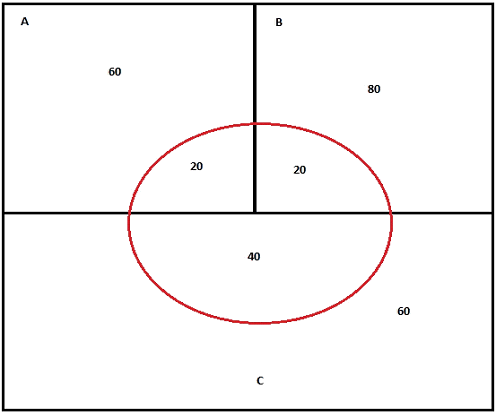1270.0.55.006 - Australian Statistical Geography Standard (ASGS): Correspondences, July 2011
Latest ISSUE Released at 11:30 AM (CANBERRA TIME) 27/06/2012  First Issue
 Page tools: .mffeedback,#pjs { display :none; } Enable Javascript to Print PagesPrint AllEmail Notification Main Features Information about the Correspondence Files About this Release History of Changes INFORMATION ABOUT THE CORRESPONDENCE FILES INTRODUCTION This publication presents a suite of geographical correspondences, primarily to assist users make comparisons and maintain time series between the previous Australian Standard Geographical Classification (ASGC) and the new Australian Statistical Geography Standard (ASGS). Correspondences are a mathematical method of reassigning data from one geographic region to another geographic region. These correspondences have been created using a new methodology. It uses a population weighted grid, representing either 2006 Census Collection Districts (CD) or 2011 Mesh Block (MB) population data, which creates far more accurate correspondences than have been previously available. While the ABS recognises that in many cases a correspondence is the only option available when attempting to convert data from one geographic region to another, caution should always be used when assessing the results of corresponded data, as they may not reflect the actual characteristics of a region. Issues surrounding the use of correspondences are discussed in the ABS publication: Information Paper: Converting Data to the Australian Statistical Geography Standard, 2011 (cat. no. 1216.0.55.004). To assist users with making a determination of how well a correspondence may or may not convert data, the ABS has developed a quality indicator which is supplied with each correspondence. This document details how the population weighted grid method produces correspondences, and provides a description of how the quality indicator is calculated. POPULATION WEIGHTED GRID CORRESPONDENCES The population weighted grid method that the ABS has adopted is essentially a series of grid points that represent the underlying geographical distribution of the weighting unit, most often CD or Mesh Block population. Each grid point is then assigned a value based on this weighting. This is demonstrated in the example below.Figure 1: Example of a CD with population grid points and SA1 regions. The correspondence in this example is from CD (the FROM region) to Statistical Area Level 1 (SA1) (the TO region), CD population is the weighting. The hypothetical CD above contains 200 persons. This population is represented by ten evenly distributed grid points, each grid point representing 20 persons. The next step in the correspondence generation process is to determine the proportion that the CD, as the FROM unit, is donating to the respective SA1 TO units. As can be seen in the diagram above there are 7 grid points in SA1 A, and three in SA1 B. Given that each grid point represents 20 persons,140 persons are located in SA1 A and 60 in SA1 B. The proportion is then calculated by dividing the population found in each of the TO regions by the total population of the FROM region. Therefore the proportions are as follow: SA1 A: 140 / 200 which gives a ratio of 0.7 or 70 per cent. SA1 B: 60 / 200 which gives a ratio of 0.3 or 30 per cent. So the result is that the CD in question is donating 70 per cent of its data to SA1 A, and 30 per cent of its data to SA1 B. The benefit of using this method is that any two sets of geographic regions can have a correspondence generated for them, and that any attribute value can be distributed across the grid to be used as the weighting unit. QUALITY INDICATOR The change in geographical classification, with the ABS moving from the ASGC to the ASGS, has resulted in an increase in demand for correspondences to convert past data to the new ASGS. As a result the ABS conducted an investigation to determine how accurately correspondences converted data. This found that while some correspondences converted data well, there were many cases where the converted data did not reflect the actual characteristics of some geographical regions. Based on these findings a quality indicator was developed to inform data users of where the converted data values are likely to be accurate, and where caution will be needed to be used when assessing the results. The method that has been developed to generate the quality indicator involves a number of steps. Firstly it looks at the value that a FROM region donates to a TO region as a ratio of the whole FROM region. The next step is to examine the value that the FROM region donates to the TO region as a ratio of the whole TO region. These two values are then multiplied together to provide the component for that FROM region. This process is then repeated for each donating FROM region, with the component values then added to provide the overall score for the TO region. Based on the score returned, a textual description is then applied as to how well the ABS expects data to be converted to the TO region. This is highlighted in the example below.Figure 2: Illustration of 3 FROM regions and 1 TO region. In this example there are three FROM regions A, B and C are represented by the black boundaries. The TO region is represented by the red ellipse. Region A donates 20 persons to the TO region, while there are a further 60 people in FROM Region A that are not donated to the TO region. Therefore the ratio of FROM region A is 20 / 80, or 0.25. The next step is to look at the value that is being donated from Region A compared to the total value of the TO region. Region A donates 20 persons, and the total population is 80. So in this case the ratio is 20 / 80, or 0.25. Region A's component score is then calculated by multiplying 0.25 x 0.25 giving Region A a component score of 0.0625. The same process is then applied to FROM Regions B and C. Region B donates 20 persons with a further 80 persons in the remainder of the FROM region. Therefore its ratio is 20 / 100 or 0.2. Region B donates 20 persons and the total population of the TO region is 80 so the ratio is 20 / 80 or 0.25. Region B's component score is therefore 0.2 x 0.25 or 0.05. Again Region C donates 40 persons with another 60 in the remainder of FROM Region C. The ratio is 40 / 100 or 0.4. The 40 persons donated are then compared against the total population of the TO region of 80, so the ratio is 40 / 80 or 0.5. This results in the component score for From Region C being 0.4 x 0.5 or 0.2. The final step is to add the three component scores. In this case: Region A = 0.0625 Region B = 0.05 Region C = 0.2 The final result is that the TO region in this example would have a quality indicator score of 0.3125, a score that the ABS would regard as being poor, meaning that caution would have to be used when using the results of data converted to the TO region. The textual descriptions and there definitions that will be supplied for each TO region in a correspondence are as follows. Good – The ABS expects that for this TO region the correspondence will convert data to a high degree of accuracy and users can expect the converted data will reflect the actual characteristics of the geographic regions involved. Acceptable – The ABS expects that for this TO region the correspondence will convert data to a reasonable degree of accuracy, though caution needs to be applied as the quality of the converted data will vary and may differ from the actual characteristics of the geographic regions involved. Poor – The ABS expects that for this TO region there is a high likelihood the correspondence will not convert data accurately and that the converted data should be used with caution as it may not reflect the actual characteristics of many of the geographic regions involved. OVERALL QUALITY INDICATOR An overall quality indicator is given to each correspondence. The aim of this is to provide users with a reasonable idea of how well the correspondence will convert data across the whole of the correspondence. The overall quality indicator is derived from multiplying the population of each TO region with that TO regions quality indicator score, based on the methodology described above. The values produced by this multiplication for each TO region are then added together. This aggregated value is then divided by the total population of the TO regions. This will return a result similar to the individual quality indicator scores. Similar textual descriptions are then applied. Good – The ABS expects that the correspondence will convert data overall to a high degree of accuracy and users can expect the converted data will reflect the actual characteristics of the geographic regions involved. Acceptable – The ABS expects that the correspondence will convert data overall to a reasonable degree of accuracy, though caution needs to be applied as the quality of the converted data will vary and may differ in parts from the actual characteristics of the geographic regions involved. Poor – The ABS expects there is a high likelihood the correspondence will not convert data overall accurately and that the converted data should be used with caution as it may not reflect the actual characteristics of many of the geographic regions involved. Document Selection These documents will be presented in a new window.